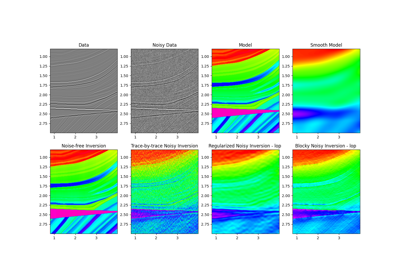# pylops.avo.poststack.PoststackInversion¶

pylops.avo.poststack.PoststackInversion(data, wav, m0=None, explicit=False, simultaneous=False, epsI=None, epsR=None, dottest=False, epsRL1=None, **kwargs_solver)[source]

Post-stack linearized seismic inversion.

Invert post-stack seismic operator to retrieve an elastic parameter of choice from band-limited seismic post-stack data. Depending on the choice of input parameters, inversion can be trace-by-trace with explicit operator or global with either explicit or linear operator.

Parameters: data : np.ndarray Band-limited seismic post-stack data of size $$[n_{t0} (\times n_x \times n_y)]$$ wav : np.ndarray Wavelet in time domain (must have odd number of elements and centered to zero). If 1d, assume stationary wavelet for the entire time axis. If 2d of size $$[n_{t0} \times n_h]$$ use as non-stationary wavelet m0 : np.ndarray, optional Background model of size $$[n_{t0} (\times n_x \times n_y)]$$ explicit : bool, optional Create a chained linear operator (False, preferred for large data) or a MatrixMult linear operator with dense matrix (True, preferred for small data) simultaneous : bool, optional Simultaneously invert entire data (True) or invert trace-by-trace (False) when using explicit operator (note that the entire data is always inverted when working with linear operator) epsI : float, optional Damping factor for Tikhonov regularization term epsR : float, optional Damping factor for additional Laplacian regularization term dottest : bool, optional Apply dot-test epsRL1 : float, optional Damping factor for additional blockiness regularization term **kwargs_solver Arbitrary keyword arguments for scipy.linalg.lstsq solver (if explicit=True and epsR=None) or scipy.sparse.linalg.lsqr solver (if explicit=False and/or epsR is not None) minv : np.ndarray Inverted model of size $$[n_{t0} (\times n_x \times n_y)]$$ datar : np.ndarray Residual data (i.e., data - background data) of size $$[n_{t0} (\times n_x \times n_y)]$$

Notes

The cost function and solver used in the seismic post-stack inversion module depends on the choice of explicit, simultaneous, epsI, and epsR parameters:

Note that the convergence of iterative solvers such as scipy.sparse.linalg.lsqr can be very slow for this type of operator. It is suggested to take a two steps approach with first a trace-by-trace inversion using the explicit operator, followed by a regularized global inversion using the outcome of the previous inversion as initial guess.

## Examples using pylops.avo.poststack.PoststackInversion¶07. Post-stack inversion